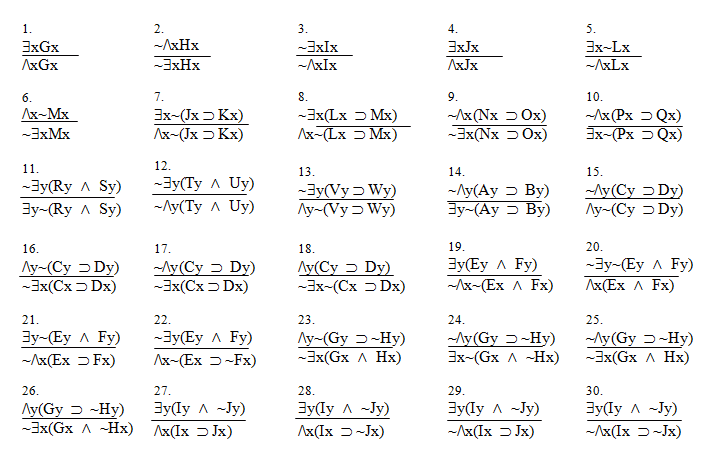Here's a rule that lets us switch between "" and "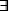" and vice versa without making any invalid inferences,

 Rule 17: Change Quantifier (CQ) Comes in eight little rules. 1. If "xP" is an available line, then "~x~P" may be written as a new line in the derivation, and 2. If "~xP" is an available line, then "x~P" may be written as a new line in the derivation, and 3. If "x~P" is an available line, then "~xP" may be written as a new line in the derivation, and 4. If "~x~P" is an available line, then "xP" may be written as a new line in the derivation, and 5. If "xP" is an available line, then "~x~P" may be written as a new line in the derivation, and 6. If "~xP" is an available line, then "x~P" may be written as a new line in the derivation, and 7. If "x~P" is an available line, then "~xP" may be written as a new line in the derivation, and 8. If "xP" is an available line, then "~x~P" may be written as a new line in the derivation.

Or, to put it another way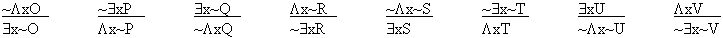Whenever an instance of the sentence above the line appears as an available line, the sentence below the line can be written.

From left to right, these arguments might say things like:

If it's not true that everything is an ostritch, then something exists that isn't an ostritch.
If it's not true that a penguin exists, then everything is a non-penguin.
If something exists that isn't a quahog, then it's not true that everything is a quahog.
If everything is a non-robot, then it's not true that a robot exists.
If it's not true that everything isn't slithy, then something exists that is slithy.
If it's not true something exists that isn't a turnip, then everything is turnips.
If an ugly thing exists, then it's not true that everything is beautiful.
If everything is vile, then it's not true that something exists that isn't vile.

Arranging things this way reveals a helpful feature of CQ. Notice that in each "argument" there are always exactly two tildes. Never more, never less. These two tilde's are either both above the line, both below, or one up and one down. Notice also that no tilde ever appears in the same position both above and below the line. There's only two places to put a tilde, and if there's a tilde in that place above the line, there isn't below the line, and vice versa. Finally, of course, the quantifier always changes. "" becomes "" and "" becomes "" every time.

Now, what happens if you apply CQ to a the result of a previous application of CQ? Well, if you apply CQ tox~P you get ~xP, right? So what happens if you apply CQ to that ~xP? (Try it. I'll wait.)

Did you getx~P? (If you didn't, try again.) Try it with another formula. Is there any formula for which two applications of CQ doesn't bring you back to the same formula? Now think about it with "Fx" instead of "P." This symmetry gives us the chance to write the meanings of the CQ rules out as a set of four equivalences, (with Fx instead of P), like so.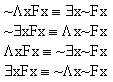You can check these by drawing pictures for each of the sides. What? You want me to draw the pictures! Why, the nerve of some people!

Okay, here's the pictures: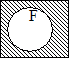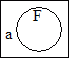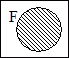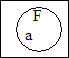Now, one of these diagrams makes both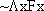andtrue, one makes both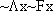and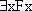true, one makes both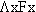and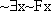true and one makes both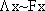andtrue. Which one is which?

On the other hand, one of these diagrams makes bothandfalse, one makes bothandfalse, one makes bothandfalse and one makes bothandfalse. Which one is which for falsity?

Now, can you find a single picture in whichandhave different truth values? Can you find a picture in whichandhave different truth values? Is there one in which haveanddifferent truth values? Or can you find a picture in whichandhave different truth values?

Here are some formulas to practice on. Which ones are justified as instances of CQ, and which ones aren't?